# Vectors and functions for space and time

The time velocity of an n-dimensional vector variable or vector-valued function Δx per unit of an independent scalar variable t equals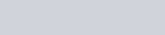Similarly, the space lenticity with Δt and Δx, respectively: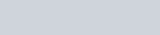The rate of a scalar variable Δx per unit of an independent n-dimensional vector variable or vector-valued function Δt equals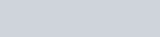The same except with Δt and Δx, respectively: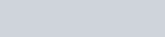The rate of an n-dimensional vector variable or vector-valued function Δx per unit of an independent n-dimensional vector variable or vector-valued function Δt equalsThe same except with Δt and Δx, respectively:The derivative of an n-dimensional vector function x with respect to an independent scalar variable t equals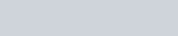The same except with t and x, respectively: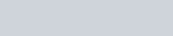The directional derivative of a scalar function x with respect to an independent n-dimensional vector variable t equals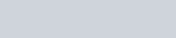The same except with t and x, respectively: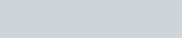The directional derivative of an n-dimensional vector-valued function x with respect to an n-dimensional vector variable t equalsThe same except with t and x, respectively:The matrices are square Jacobian matrices, whose (i, j)th entry is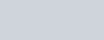The inverse function theorem states that the matrix inverse of the Jacobian matrix of an invertible function is the Jacobian matrix of the inverse function.